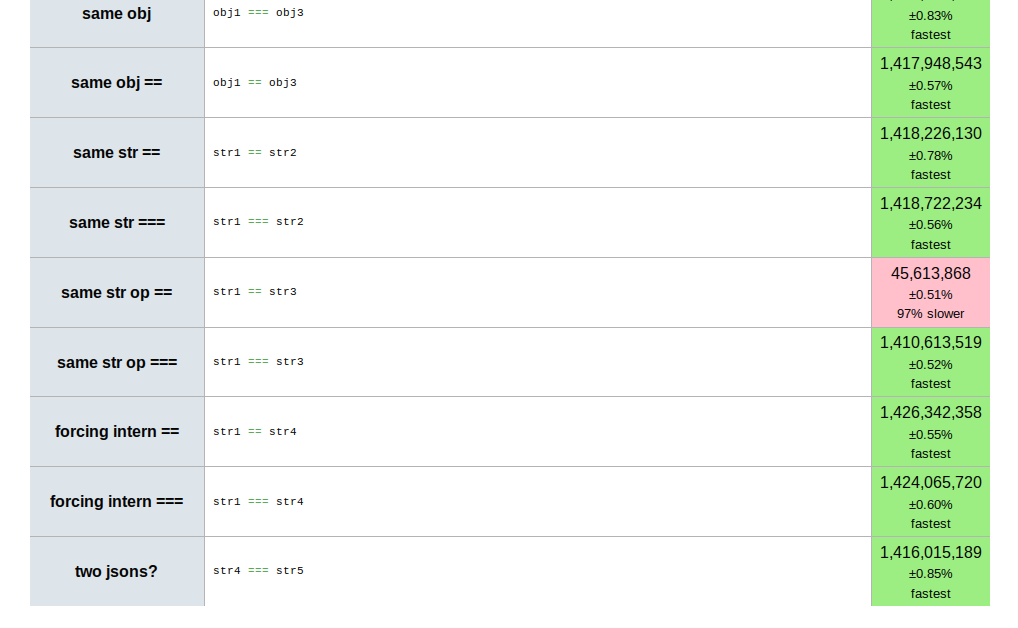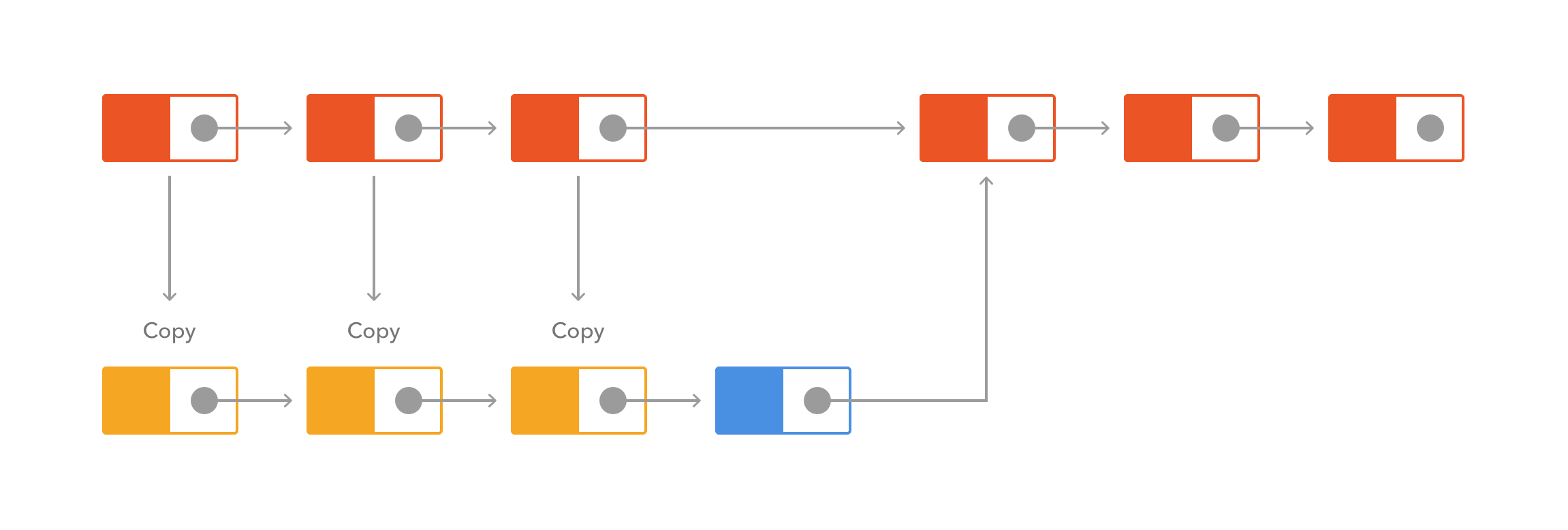# [译] Immutable.js 与函数式编程概念介绍

2016-07-240 篇评论

## 介绍：不可变（immutability）和 Immutable.js## 核心内容

### 不可变

``````var list1 = Immutable.List.of(1, 2);
// 我们需要通过返回值来获取结果：
// list1 没有被修改！
var list2 = list1.push(3, 4, 5);
``````

• 为多线程加锁不再是一个问题：当数据不可变时，再也不需要使用锁来保持多线程的同步了。
• 持久化（下面将提到的一个重要概念）变得容易。
• 拷贝变成了一个常量时间的操作：拷贝只是简单的为已存在的数据结构创建一个新的引用。
• 值比较在特定的情况下可以被简化：此时运行时或编译器能确保，一个特定的实例只有在指向相同的引用时才会是相等的，便能通过引用比较来替代深层比较。这通常只可用在编译或者加载时数据可用的情况下。这样的优化也可以被手动控制（React 和 Angular 就是这样做的，在文章末尾会做出解释）

#### 你已经在使用一个不可变数据结构：String#### 不可变与 Object.freeze()

Javascript 是一个动态、弱类型的语言（或者是无类型，如果你熟悉编程语言理论的话）。正因如此，有时难以强制对对象和数据的某些约束。`Object.freeze()` 帮我们解决了这个问题。对 `Object.freeze` 的调用标志着这个对象的所有属性都成为不可变的。对它的修改要么不成功，要么抛出一个异常（在严格模式下）。如果你正写一个不可变对象，在完成初始化之后调用 `Object.freeze` 能帮上忙。

``````function deepFreeze(obj) {
// 取出这个对象中的所有 property
var propNames = Object.getOwnPropertyNames(obj);

// 在冻结自己之前，先把所有的 property 冻结
propNames.forEach(function(name) {
var prop = obj[name];

// 如果是一个 object，则冻结
if (typeof prop == 'object' && prop !== null) {
deepFreeze(prop);
}
});

// 冻结自己（如果已被冻结则什么也不会发生）
return Object.freeze(obj);
}
``````

### 副作用

``````var globalCounter = 99;

// 这个函数改变了全局状态
function add(a, b) {
++globalCounter;
return a + b;
}

// 上面那个看起来无辜的 add 函数调用可能会对
// 这个控制台的打印结果产生异常的修改
function printCounter() {
console.log(globalCounter.toString());
}
``````

#### 纯

``````var globalValue = 99;

// 这个函数是不纯的：如果 globalValue 改变，它的结果也会发生改变，
// 即使传入的值 a 和 b 与之前的相同。
function sum(a, b) {
return a + b + globalValue;
}
``````

#### 引用透明

``````function add(a, b) {
return a + b;
}

// 下面的调用能被它的结果 3 所取代。因为它的引用透明让这样成为可能。换句话说，它是无副作用的纯函数
var r1 = add(1, 2); // r1 = 3;
``````

### 持久化（Persistence）

``````var list1 = Immutable.List.of(1, 2);
var list2 = list1.push(3, 4, 5);
var list3 = list2.unshift(0);
var list4 = list1.concat(list2, list3);
``````

### 惰性求值

``````var oddSquares = Immutable.Seq.of(1,2,3,4,5,6,7,8)
.filter(x => x % 2)
.map(x => x * x);
// 只有在需要获取结果的时候才会进行计算操作
console.log(oddSquares.get(1)); // 9
``````

``````function plusOne(n) {
return n + 1;
}
function plusTen(n) {
return n + 10;
}

var list = [1,2,3,4,5,6,7,8,9,10];
var result = list.map(plusOne).map(plusTen);
``````

### 组合

``````Immutable.Range(1, Infinity)
.skip(1000)
.map(n => -n)
.filter(n => n % 2 === 0)
.take(2)
.reduce((r, n) => r * n, 1);
``````

### 备用方式：变化

``````var list1 = Immutable.List.of(1,2,3);
var list2 = list1.withMutations(function (list) {
list.push(4).push(5).push(6);
});
``````

## 算法考虑• 头部插入：O(1)
• 尾部插入：O(1)
• 中间插入：O(1)
• 查找: O(n)
• 拷贝：O(n)

• 头部插入：O(1)
• 尾部插入：O(n)
• 中间插入：O(n)
• 查找：O(n)
• 拷贝：O(1)

## 示例：React DBMon 跑分

``````// 通过跳过一些更新来测试重新渲染状态检查
var skip = Math.random() >= 0.25;

Object.keys(newData.databases).forEach(function (dbname) {
if (skip) {
return;
}

//(...)
});
``````

``````if (!this.state.databases[dbname]) {
this.state.databases[dbname] = {
name: dbname,
samples: Immutable.List()
};
}

this.state.databases[dbname].samples =
this.state.databases[dbname].samples.push({
time: newData.start_at,
queries: sampleInfo.queries
});
if (this.state.databases[dbname].samples.size > 5) {
this.state.databases[dbname].samples =
this.state.databases[dbname].samples.skip(
this.state.databases[dbname].samples.size - 5);
}
``````
``````var Database = React.createClass({
displayName: "Database",

mixins: [React.PureRenderMixin],

render: function render() {
//(...)
}
//(...)
});
``````（必填）

（必填）

（选填）